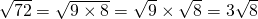# Estimate Square Roots

Now because we are familiar with, we can figure the two integers a square root (from zero to 144) lies between.

For example,lies between 5 and 6, becauseand.

# Simplifying a surd

Often times a non-square number under a square root (radicand) can be written as a product of a square number by a non-square number. This is called simplifying the surd.

First, you need to factor the radicand. We’re looking for the highest factor that is a perfect square.

The first 12 positive square numbers are:In the applet below, think of the highest square number that will divide (is a factor of) the integer given.

Example: SimplifyLet’s list the factors of 72.

Here are the factors: 1, 2, 3, 4, 6, 8, 9, 12, 18, 24, 36, 72

The factors that are also perfect squares are: 4, 9, 36 (also 1 but 1 is not useful here).

The highest is 36.

So,Suppose I selected 9 or 4 because I didn’t spot that 36 was a factor. In this case, I end up with another surd that can be simplified, so I just simplify again.

e.g.andTherefore,Simplify the square roots by writing as a product of a square number with a non square number:

# Rationalise a denominator

It is considered ‘more simple’ to multiply by an irrational number than to divide by one. Suppose we have the fraction:We can rationalise the denominator by multiplying this fraction by 1, whereas follows:We end up with a rational number on the denominator, which was the goal. This is often used to simplify lines of work in trigonometry.Here’s another trick of numbers to simplify an irrational denominator. Suppose we have the fractionWe can rationalise this denominator by multiplying this fraction by 1, whereas follows:Again, our denominator is now rational.

Try the ten questions at the bottom of this mathisfun page!